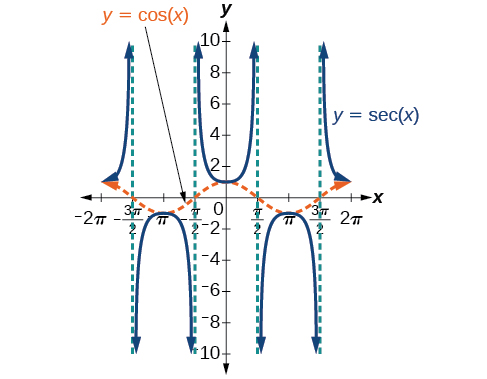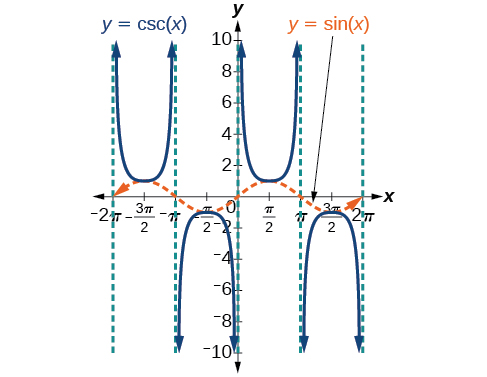8.2 Graphs of the other trigonometric functions  (Page 4/9)

 Page 4 / 9

The secant graph has vertical asymptotes at each value of $\text{\hspace{0.17em}}x\text{\hspace{0.17em}}$ where the cosine graph crosses the x -axis; we show these in the graph below with dashed vertical lines, but will not show all the asymptotes explicitly on all later graphs involving the secant and cosecant.

Note that, because cosine is an even function, secant is also an even function. That is, $\text{\hspace{0.17em}}\mathrm{sec}\left(-x\right)=\mathrm{sec}\text{\hspace{0.17em}}x.$Graph of the secant function,   f ( x ) = sec x = 1 cos x

As we did for the tangent function, we will again refer to the constant $\text{\hspace{0.17em}}|A|\text{\hspace{0.17em}}$ as the stretching factor, not the amplitude.

Features of the graph of y = A Sec( Bx )

• The stretching factor is $\text{\hspace{0.17em}}|A|.$
• The period is $\text{\hspace{0.17em}}\frac{2\pi }{|B|}.$
• The domain is $\text{\hspace{0.17em}}x\ne \frac{\pi }{2|B|}k,\text{\hspace{0.17em}}$ where $\text{\hspace{0.17em}}k\text{\hspace{0.17em}}$ is an odd integer.
• The range is $\text{\hspace{0.17em}}\left(-\infty ,-|A|\right]\cup \left[|A|,\infty \right).$
• The vertical asymptotes occur at $\text{\hspace{0.17em}}x=\frac{\pi }{2|B|}k,\text{\hspace{0.17em}}$ where $\text{\hspace{0.17em}}k\text{\hspace{0.17em}}$ is an odd integer.
• There is no amplitude.
• $y=A\mathrm{sec}\left(Bx\right)\text{\hspace{0.17em}}$ is an even function because cosine is an even function.

Similar to the secant, the cosecant    is defined by the reciprocal identity $\text{\hspace{0.17em}}\mathrm{csc}\text{\hspace{0.17em}}x=\frac{1}{\mathrm{sin}\text{\hspace{0.17em}}x}.\text{\hspace{0.17em}}$ Notice that the function is undefined when the sine is 0, leading to a vertical asymptote in the graph at $\text{\hspace{0.17em}}0,\text{\hspace{0.17em}}$ $\pi ,\text{\hspace{0.17em}}$ etc. Since the sine is never more than 1 in absolute value, the cosecant, being the reciprocal, will never be less than 1 in absolute value.

We can graph $\text{\hspace{0.17em}}y=\mathrm{csc}\text{\hspace{0.17em}}x\text{\hspace{0.17em}}$ by observing the graph of the sine function because these two functions are reciprocals of one another. See [link] . The graph of sine is shown as a dashed orange wave so we can see the relationship. Where the graph of the sine function decreases, the graph of the cosecant function increases. Where the graph of the sine function increases, the graph of the cosecant function decreases.

The cosecant graph has vertical asymptotes at each value of $\text{\hspace{0.17em}}x\text{\hspace{0.17em}}$ where the sine graph crosses the x -axis; we show these in the graph below with dashed vertical lines.

Note that, since sine is an odd function, the cosecant function is also an odd function. That is, $\text{\hspace{0.17em}}\mathrm{csc}\left(-x\right)=\mathrm{-csc}x.$

The graph of cosecant, which is shown in [link] , is similar to the graph of secant.The graph of the cosecant function,   f ( x ) = csc x = 1 sin x

Features of the graph of y = A Csc( Bx )

• The stretching factor is $\text{\hspace{0.17em}}|A|.$
• The period is $\text{\hspace{0.17em}}\frac{2\pi }{|B|}.$
• The domain is $\text{\hspace{0.17em}}x\ne \frac{\pi }{|B|}k,\text{\hspace{0.17em}}$ where $\text{\hspace{0.17em}}k\text{\hspace{0.17em}}$ is an integer.
• The range is $\left(-\infty ,-|A|\right]\cup \left[|A|,\infty \right).$
• The asymptotes occur at $\text{\hspace{0.17em}}x=\frac{\pi }{|B|}k,\text{\hspace{0.17em}}$ where $\text{\hspace{0.17em}}k\text{\hspace{0.17em}}$ is an integer.
• $y=A\mathrm{csc}\left(Bx\right)\text{\hspace{0.17em}}$ is an odd function because sine is an odd function.

Graphing variations of y = sec x And y = csc x

For shifted, compressed, and/or stretched versions of the secant and cosecant functions, we can follow similar methods to those we used for tangent and cotangent. That is, we locate the vertical asymptotes and also evaluate the functions for a few points (specifically the local extrema). If we want to graph only a single period, we can choose the interval for the period in more than one way. The procedure for secant is very similar, because the cofunction identity means that the secant graph is the same as the cosecant graph shifted half a period to the left. Vertical and phase shifts may be applied to the cosecant function in the same way as for the secant and other functions.The equations become the following.

A laser rangefinder is locked on a comet approaching Earth. The distance g(x), in kilometers, of the comet after x days, for x in the interval 0 to 30 days, is given by g(x)=250,000csc(π30x). Graph g(x) on the interval [0, 35]. Evaluate g(5)  and interpret the information. What is the minimum distance between the comet and Earth? When does this occur? To which constant in the equation does this correspond? Find and discuss the meaning of any vertical asymptotes.
The sequence is {1,-1,1-1.....} has
how can we solve this problem
Sin(A+B) = sinBcosA+cosBsinA
Prove it
Eseka
Eseka
hi
Joel
June needs 45 gallons of punch. 2 different coolers. Bigger cooler is 5 times as large as smaller cooler. How many gallons in each cooler?
7.5 and 37.5
Nando
find the sum of 28th term of the AP 3+10+17+---------
I think you should say "28 terms" instead of "28th term"
Vedant
the 28th term is 175
Nando
192
Kenneth
if sequence sn is a such that sn>0 for all n and lim sn=0than prove that lim (s1 s2............ sn) ke hole power n =n
write down the polynomial function with root 1/3,2,-3 with solution
if A and B are subspaces of V prove that (A+B)/B=A/(A-B)
write down the value of each of the following in surd form a)cos(-65°) b)sin(-180°)c)tan(225°)d)tan(135°)
Prove that (sinA/1-cosA - 1-cosA/sinA) (cosA/1-sinA - 1-sinA/cosA) = 4
what is the answer to dividing negative index
In a triangle ABC prove that. (b+c)cosA+(c+a)cosB+(a+b)cisC=a+b+c.
give me the waec 2019 questionsByByBy Lakeima RobertsBy Anindyo MukhopadhyayBy